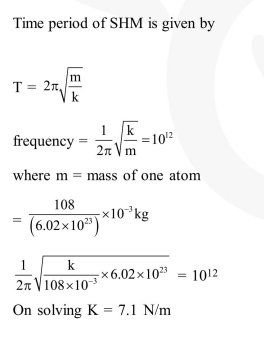# A silver atom in a solid oscillates in simple harmonic motion in some direction`
Question:

A silver atom in a solid oscillates in simple harmonic motion in some direction with a frequency of $10^{12} / \mathrm{sec}$. What is the force constant of the bonds connecting one atom with the other ? (Mole wt. of silver $=108$ and Avagadro number $=6.02 \times 10^{23} \mathrm{gm} \mathrm{mole}^{-1}$ )

1. $7.1 \mathrm{~N} / \mathrm{m}$

2. $2.2 \mathrm{~N} / \mathrm{m}$

3. $5.5 \mathrm{~N} / \mathrm{m}$

4. $6.4 \mathrm{~N} / \mathrm{m}$

Correct Option: 1

Solution: